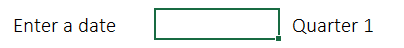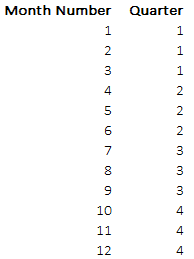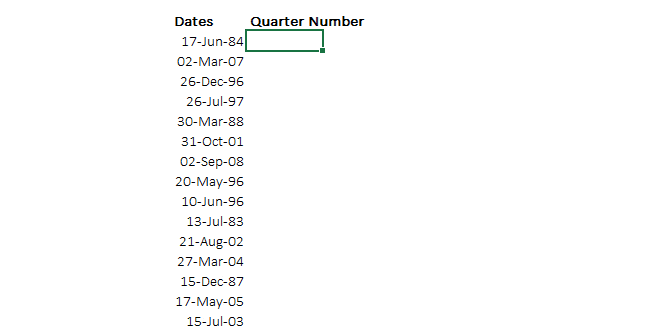I am dedicating this post to my brother, who asked me a simple question last night to find out quarters for a given set of dates. For example

• 02-Feb-2014 is the 1st Quarter
• 15-Apr-2013 is the 2nd Quarter
• 27-Aug-2010 is the 3rd Quarter

I have 2 ways to solve this

#### 1. The Vlookup Way

Consider these dates for which you need to find quartersNow let’s create a helper table some where on the sheet including month number (Jan stand for 1, Feb for 2 and so on till Dec) and their quarters.. something like thisNow as simple as that .. Apply the VLOOKUP on your dates [Learn VLOOKUP + some awesome tricks]Note 2 things here

• I am using the MONTH function on the date to first extract the month number. =Month(any date) will give you the month number (starting from Jan as 1)

#### 2. The Formula Way

We can solve this with a formula too. Take a look.. not too tricky trust me !

1. Use the MONTH function on the date to extract the month
2. Wrap the MONTH function in the =CEILING function, this is how the CEILING function works
• =CEILING(number,significance)
• The number could be any number, in this case its is our month number
• Significance is the multiple, so if I write =CEILING(5,3) the result will be 6, it will simply push the number 5 to the next multiple of 3
• In our case Significance is 3 (logic: since each quarter is made of 3 months), so you’ll have results as 3,6,9 or 12 for any month
3. Now wrap the CEILING formula into a QUOTIENT formula, this is how it works
• =QUOTIENT(numerator, denominator)
• I think the syntax makes it pretty clear, the QUOTIENT function returns the quotient for the numerator and denominator specified
• In our case the numerator will be the multiple obtained by the CEILING function (3,6,9,12) and denominator will be 3
• So the output (quotient) will be either quarter 1 ,2,3 or 4

Take a look at the formula construct =QUOTIENT(CEILING(MONTH(date),3),3)#### Now it gets a bit tricky here ! (but not too much)

Accountants and analysts can say that, my financial year ends in March (that is the way it happens in India), so we need to tweak the logic a bit

###### the logic for March ending financial year – move the dates 3 months priorThat is how the formulas should pick up the quarters !

let’s try and tame that too.. 😀

1. If my date is 10 Jan 2014, then according to March ending financial year my quarter is 4th and not 1st
2. Let’s use the EOMONTH function to move the dates 3 months prior. So =EOMONTH(“10-Jan-2010”,-3) this will return you a 3 months prior date i.e. 31 Oct  2013. Note: The EOMONTH function returns a month ending date (prior or in future)
3. Now this date (31-Oct-2013) falls under the 4th quarter. Now you can wrap the EOMONTH in, MONTH, CEILING and the QUOTIENT functions to get the correct quarters

Take a look and the formula construct =QUOTIENT(CEILING(MONTH(EOMONTH(date,-3)),3),3)How do you work around with finding quarters with dates in excel ? Share your tricks using comments